## Solve Differential Algebraic Equations (DAEs)

### What is a Differential Algebraic Equation?

Differential algebraic equations are a type of differential equation where one or more derivatives of dependent variables are not present in the equations. Variables that appear in the equations without their derivative are called algebraic, and the presence of algebraic variables means that you cannot write down the equations in the explicit form $y\text{'}=f\left(t,y\right)$. Instead, you can solve DAEs with these forms:

• The ode15s and ode23t solvers can solve index-1 linearly implicit problems with a singular mass matrix $M\left(t,y\right)y\text{'}=f\left(t,y\right)$, including semi-explicit DAEs of the form

$\begin{array}{c}y\text{'}=f\left(t,y,z\right)\\ 0=g\left(t,y,z\right)\text{\hspace{0.17em}}.\end{array}$

In this form, the presence of algebraic variables leads to a singular mass matrix, since there are one or more zeros on the main diagonal.

$My\text{'}=\left(\begin{array}{cccc}y{\text{'}}_{1}& 0& \cdots & 0\\ 0& y{\text{'}}_{2}& 0& ⋮\\ ⋮& 0& \ddots & 0\\ 0& \cdots & 0& 0\end{array}\right)\text{\hspace{0.17em}}.$

By default, solvers automatically test the singularity of the mass matrix to detect DAE systems. If you know about singularity ahead of time then you can set the MassSingular option of odeset to 'yes'. With DAEs, you can also provide the solver with a guess of the initial conditions for $y{\text{'}}_{0}$ using the InitialSlope property of odeset. This is in addition to specifying the usual initial conditions for ${y}_{0}$ in the call to the solver.

• The ode15i solver can solve more general DAEs in the fully implicit form

$f\left(t,y,y\text{'}\right)=0\text{\hspace{0.17em}}.$

In the fully implicit form, the presence of algebraic variables leads to a singular Jacobian matrix. This is because at least one of the columns in the matrix is guaranteed to contain all zeros, since the derivative of that variable does not appear in the equations.

$J=\partial f/\partial y\text{'}=\left(\begin{array}{ccc}\frac{\partial {f}_{1}}{\partial y{\text{'}}_{1}}& \cdots & \frac{\partial {f}_{1}}{\partial y{\text{'}}_{n}}\\ ⋮& \ddots & ⋮\\ \frac{\partial {f}_{m}}{\partial y{\text{'}}_{1}}& \cdots & \frac{\partial {f}_{m}}{\partial y{\text{'}}_{n}}\end{array}\right)$

The ode15i solver requires that you specify initial conditions for both $y{\text{'}}_{0}$ and ${y}_{0}$. Also, unlike the other ODE solvers, ode15i requires the function encoding the equations to accept an extra input: odefun(t,y,yp).

DAEs arise in a wide variety of systems because physical conservation laws often have forms like $x+y+z=0$. If x, x', y, and y' are defined explicitly in the equations, then this conservation equation is sufficient to solve for z without having an expression for z'.

### Consistent Initial Conditions

When you are solving a DAE, you can specify initial conditions for both $y{\text{'}}_{0}$ and ${y}_{0}$. The ode15i solver requires both initial conditions to be specified as input arguments. For the ode15s and ode23t solvers, the initial condition for $y{\text{'}}_{0}$ is optional (but can be specified using the InitialSlope option of odeset). In both cases, it is possible that the initial conditions you specify do not agree with the equations you are trying to solve. Initial conditions that conflict with one another are called inconsistent. The treatment of the initial conditions varies by solver:

• ode15s and ode23t — If you do not specify an initial condition for $y{\text{'}}_{0}$, then the solver automatically computes consistent initial conditions based on the initial condition you provide for ${y}_{0}$. If you specify an inconsistent initial condition for $y{\text{'}}_{0}$, then the solver treats the values as guesses, attempts to compute consistent values close to the guesses, and continues on to solve the problem.

• ode15i — The initial conditions you supply to the solver must be consistent, and ode15i does not check the supplied values for consistency. The helper function decic computes consistent initial conditions for this purpose.

### Differential Index

DAEs are characterized by their differential index, which is a measure of their singularity. By differentiating equations you can eliminate algebraic variables, and if you do this enough times then the equations take the form of a system of explicit ODEs. The differential index of a system of DAEs is the number of derivatives you must take to express the system as an equivalent system of explicit ODEs. Thus, ODEs have a differential index of 0.

An example of an index-1 DAE is

$y\left(t\right)=k\left(t\right)\text{\hspace{0.17em}}.$

For this equation, you can take a single derivative to obtain the explicit ODE form

$y\text{'}=k\text{'}\left(t\right)\text{\hspace{0.17em}}.$

An example of an index-2 DAE is

$\begin{array}{l}y{\text{'}}_{1}={y}_{2}\\ 0=k\left(t\right)-{y}_{1}\text{\hspace{0.17em}}.\end{array}$

These equations require two derivatives to be rewritten in the explicit ODE form

$\begin{array}{l}y{\text{'}}_{1}=k\text{'}\left(t\right)\\ y{\text{'}}_{2}=k\text{'}\text{'}\left(t\right)\text{\hspace{0.17em}}.\end{array}$

The ode15s and ode23t solvers only solve DAEs of index 1. If the index of your equations is 2 or higher, then you need to rewrite the equations as an equivalent system of index-1 DAEs. It is always possible to take derivatives and rewrite a DAE system as an equivalent system of index-1 DAEs. Be aware that if you replace algebraic equations with their derivatives, then you might have removed some constraints. If the equations no longer include the original constraints, then the numerical solution can drift.

If you have Symbolic Math Toolbox™, then see Solve Differential Algebraic Equations (DAEs) (Symbolic Math Toolbox) for more information.

### Imposing Nonnegativity

Most of the options in odeset work as expected with the DAE solvers ode15s, ode23t, and ode15i. However, one notable exception is with the use of the NonNegative option. The NonNegative option does not support implicit solvers (ode15s, ode23t, ode23tb) applied to problems with a mass matrix. Therefore, you cannot use this option to impose nonnegativity constraints on a DAE problem, which necessarily has a singular mass matrix. For more details, see .

### Solve Robertson Problem as Semi-Explicit Differential Algebraic Equations (DAEs)

This example reformulates a system of ODEs as a system of differential algebraic equations (DAEs). The Robertson problem found in hb1ode.m is a classic test problem for programs that solve stiff ODEs. The system of equations is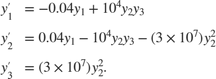hb1ode solves this system of ODEs to steady state with the initial conditions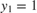,, and. But the equations also satisfy a linear conservation law,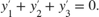In terms of the solution and initial conditions, the conservation law is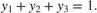The system of equations can be rewritten as a system of DAEs by using the conservation law to determine the state of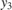. This reformulates the problem as the DAE systemThe differential index of this system is 1, since only a single derivative ofis required to make this a system of ODEs. Therefore, no further transformations are required before solving the system.

The function robertsdae encodes this DAE system. Save robertsdae.m in your current folder to run the example.

function out = robertsdae(t,y) out = [-0.04*y(1) + 1e4*y(2).*y(3) 0.04*y(1) - 1e4*y(2).*y(3) - 3e7*y(2).^2 y(1) + y(2) + y(3) - 1 ]; 

The full example code for this formulation of the Robertson problem is available in hb1dae.m.

Solve the DAE system using ode15s. Consistent initial conditions for y0 are obvious based on the conservation law. Use odeset to set the options:

• Use a constant mass matrix to represent the left hand side of the system of equations.• Set the relative error tolerance to 1e-4.

• Use an absolute tolerance of 1e-10 for the second solution component, since the scale varies dramatically from the other components.

• Leave the 'MassSingular' option at its default value 'maybe' to test the automatic detection of a DAE.

y0 = [1; 0; 0]; tspan = [0 4*logspace(-6,6)]; M = [1 0 0; 0 1 0; 0 0 0]; options = odeset('Mass',M,'RelTol',1e-4,'AbsTol',[1e-6 1e-10 1e-6]); [t,y] = ode15s(@robertsdae,tspan,y0,options); 

Plot the solution.

y(:,2) = 1e4*y(:,2); semilogx(t,y); ylabel('1e4 * y(:,2)'); title('Robertson DAE problem with a Conservation Law, solved by ODE15S');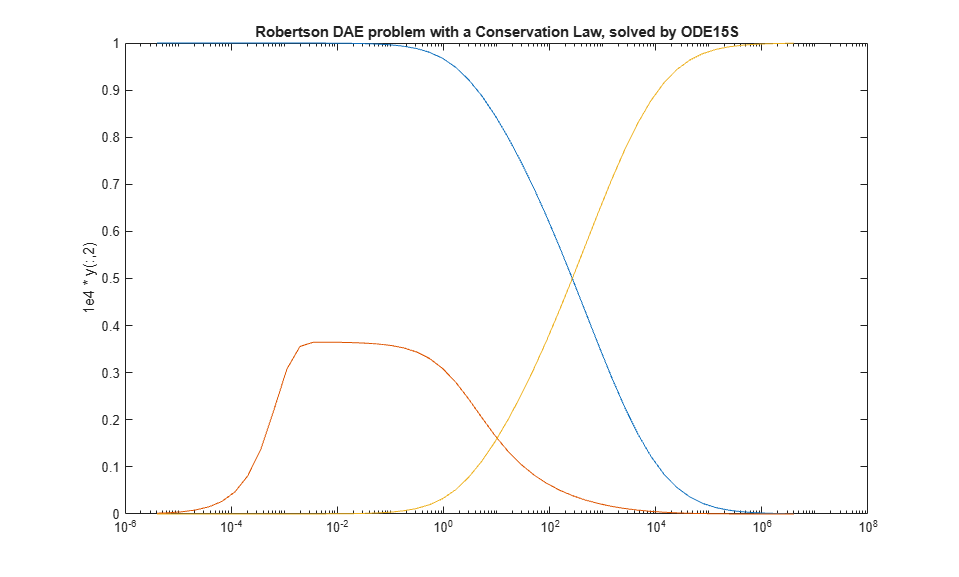Shampine, L.F., S. Thompson, J.A. Kierzenka, and G.D. Byrne. “Non-Negative Solutions of ODEs.” Applied Mathematics and Computation 170, no. 1 (November 2005): 556–569. https://doi.org/10.1016/j.amc.2004.12.011.